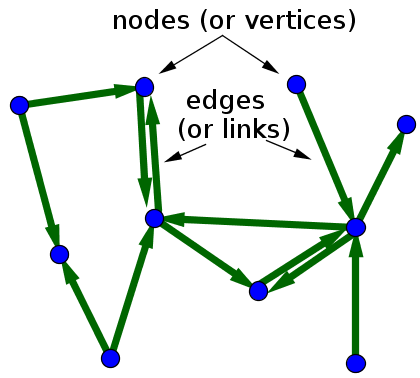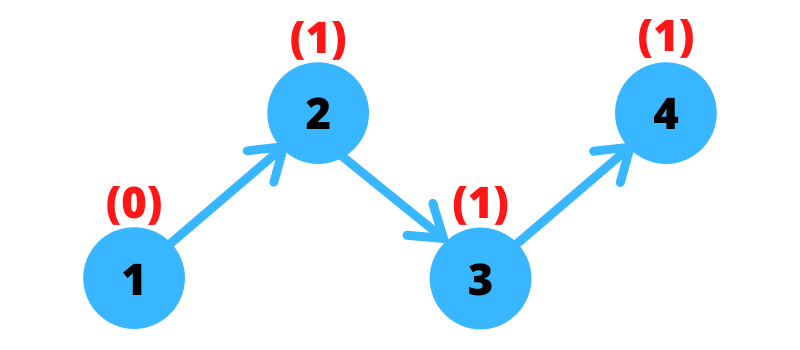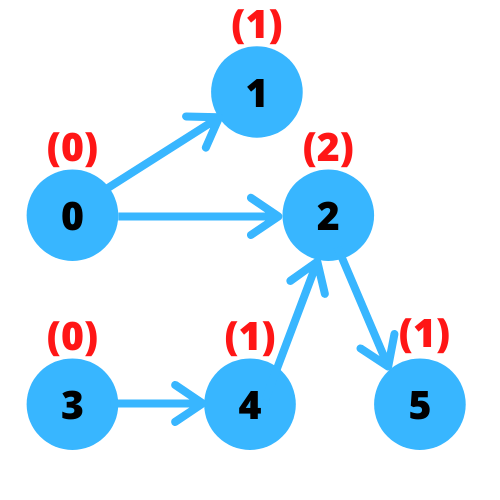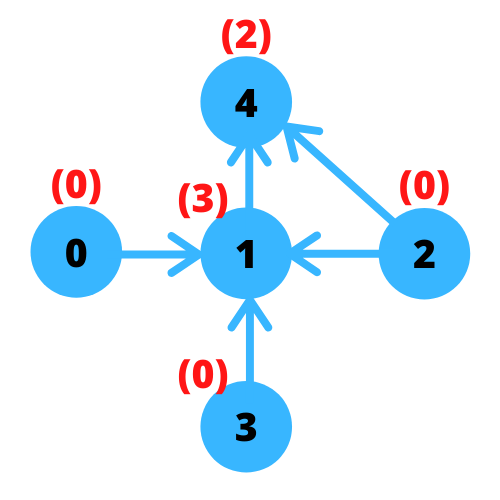# Minimum Number of Vertices to Reach All Nodes by Graph Theory with Python and Javascript

Created on Oct 6, 2020
Updated on Oct 7, 2020

## Understanding the Problem

Minimum Number of Vertices to Reach All Nodes is a medium problem at leetcode, I hope you have read the problem carefully and have tried solving it. This problem requires you to get the least number of vertices that can make you pass all nodes you have for the graph of your problem. The question is what are these vertices?

## Why graph theory?

You’re given an array of arrays (or a list of lists in a pythonic way) and each subarray contains two numbers entailing the first one to be the node from which you can move and the other one is the node to which you reach. These nodes are called vertices and each path connecting them is called an edge, the whole picture is a graph. In mathematics, graph theory is a study of such graphs and in this problem, we have a specific type of graph called directed graph where edges are connecting vertices asymmetrically meaning all edges are connecting from one vertex to another as you can see from the arrows in the image below:A directed graph with 10 vertices and 13 edges ( source )

## How graph works in this problem?

To know how it works, we need to know one thing: in-degree. Let’s see what that means through these examples

### Example 1

Say we have this simple example 1 -> 2 ->3 -> 4 as shown in the image below:author image

To reach all nodes we need to start from the beginning (I mean from node 1). Say we started from 2, we then left 1 not taken. If we start from 3, we leave 1 and 2 behind, so in order to reach all nodes with a minimum number of vertices, we need to have a node that is not having edges coming into it like 1 here. What does that mean in graph theory? We need to know the in-degree of each vertex which is the number of edges coming in each node. I highlighted the in-degree as red above each node so as node 1 having zero in-degree that means there are no edges coming in node 1. We have 1 edge coming to 2 so the in-degree of node 2 is 1. Hence, in-degree(node 3) = 1 and in-degree(node 4) = 1. That’s why 1 here is the vertex that can pass all nodes.

### Example 2

In the previous example, we didn’t actually get into the minimum number of vertices that can pass all nodes because we just had one vertex (node 1). Considering here another example from leetcode will show us the big picture of this problem. Example here: 0 -> 1, 0 -> 2, 3 -> 4 -> 2 -> 5 as shown below:author image

It’s more clear now the importance of computing the in-degree of each node especially because we have now two paths starting from 0 and another path starting from 3. We can notice that the in-degree of 0 and 3 is 0 because there are no edges coming into either of them.

### Example 3

Here we’re having 5 nodes:author image

It seems that the destination is node 4. If we pick 0 we will get to 1 and 4, we will then need to pick 2 and 3 to make us pass all nodes. That’s why 0, 2, and 3 are the minimum number of vertices that can reach all nodes because each in-degree is zero

## More problem-solving?

If you want to see more problem-solving blog posts, check out:

https://www.ezzeddinabdullah.com/categories/problem-solving